## Work, Energy and Power MCQ for NEET

NEET  Physics is the scoring paper in the medical entrance examination. Here, you will discover the NEET Physics MCQ Questions for all Concepts as per the latest syllabus. Practice more on a regular basis with these NEET Physics objective questions on air pollution and improve your subject knowledge & problem-solving skills along with time management. NEET Physics Work, Energy and Power Multiple Choice Questions make you feel confident in answering the question in the exam & increases your scores to high.

## MCQ on Work, Energy and Power

1. By how much does kinetic energy increase if the momentum is increased by 20%
(a) 55%
(b) 66%
(c) 44%
(d) 77%

2. Calculate the energy loss in a perfectly inelastic collision if the mass of the object is 40kg with velocity 4m/s hits the object of mass 60kg with velocity 2m/s.
(a) 440J
(b) 110J
(c) 392J
(d) 48J

3. Calculate the final kinetic energy of a block of mass if the mass of the block is 10kg and has a constant velocity of 10m/s. The block of mass is subjected to a retarding force of F=0.1J/m
(a) 275J
(b) 250J
(c) 475J
(d) 450J

4. What is the average power required to lift a mass of 100kg to a height of 50m in 50 seconds?
(a) 980
(b) 100
(c) 50
(d) 5000

5. What is the power of the engine when the velocity of the car is v, mass m, acceleration a, and external resistance R
(a) (R-ma)v
(b) (R+ma)v
(c) mav
(d) Rv

6. Consider an object with m as its mass such that it is accelerated uniformly from rest and the speed attained by the object is v in T time.  Calculate the instantaneous power that is delivered to the body in terms of function of time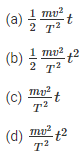Answer: (b) $$\frac{1}{2} \frac{m v^{2}}{T^{2}} t^{2}$$

7. A ball is thrown from a height of 20 m vertically downward and has an initial velocity of $$v_{0}^{2}$$. As the stone collides with the ground 50% of its energy is lost and bounce back to the same height. What is the initial velocity?
(a) 28m/s
(b) 20m/s
(c) 10m/s
(d) 14m/s

8. Consider a particle of mass m which is driven by a machine that has a constant power k Watts. What is the force on the particle at time t if the particle starts from the rest?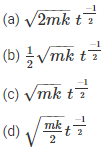Answer: (d) $$\sqrt{\frac{m k}{2}} t^{\frac{-1}{2}}$$

9. When can one say that work is done on the body
(a) When the body experiences force
(b) When there is an increase in energy because of mechanical influence
(c) When the body moves a certain distance
(d) None of the above

Answer: (b) When there is an increase in energy because of mechanical influence

10. Select the incorrect statement for the given situation: Two bodies with mass m1 and m2 undergo elastic collision
(a) When m1 and m2 is at rest, the kinetic energy transferred will be maximum
(b) The bodies will move in the same direction if m1 = m2 and when the collision is in oblique
(c) When m> m2, m2 will move with four times increase in its velocity than m1
(d) When m1 < m2, the transfer of momentum will be maximum

Answer: (c) When m1 > m2, m2 will move with four times increase in its velocity than m1

11. What is the ratio of velocities of a nucleus that splits into two parts with radii 1:2
(a) 6:1
(b) 2:1
(c) 8:1
(d) 4:1

12. What is the relation between E, P, and E if E is kinetic energy, P is momentum, and V is the velocity of the particleAnswer: (d) $$P=\frac{d E}{d V}$$

## Gravitation MCQs for NEET

NEET  Physics is the scoring paper in the medical entrance examination. Here, you will discover the NEET Physics MCQ Questions for all Concepts as per the latest syllabus. Practice more on a regular basis with these NEET Physics objective questions on air pollution and improve your subject knowledge & problem-solving skills along with time management. NEET Physics Gravitation Multiple Choice Questions make you feel confident in answering the question in the exam & increases your scores to high.

## MCQ on Gravitation

1. What happens to the gravitational potential at the centre of the uniform spherical shell which shrinks gradually?
(a) Remains constant
(b) Decreases
(c) Increases
(d) Oscillates

2. What is the relation between the escape velocity and orbital velocity of a satellite, if the satellite is close to the earth’s surface?Answer: (b) $$v_{e}=\sqrt{2} v_{0}$$

3. What happens to the weight of the body if the weight becomes $$\frac{1}{16}$$ at a certain. Also, consider the radius of the earth to be R.
(a) 4R
(b) 15R
(c) 5R
(d) 3R

4. What is the maximum height attained by the rocket above the surface of the earth if the mass of the rocket is M, and the initial speed to be V. Assume that R is the radius of the earth.Answer: (b) $$\frac{R}{\frac{2 g R}{v^{2}}-1}$$

5. What is the increase in the potential energy of the body with mass m if the body is taken at the height h which is equal to the radius of the earth?
(a) mgR
(b) 2mgR
(c) $$\frac{1}{2}$$mgR
(d) $$\frac{1}{4}$$mgR

Answer: (c) $$\frac{1}{2}$$mgR

6. Consider a body of mass m which needs to be moved from an orbit of radius 2R to 3R. What is the energy requiredAnswer: (b) $$\frac{G M m}{6 R}$$

7. The distance between the closest planet and the sun is r1 while the distance between the farthest planet and the sun is r2. The linear velocities of these planets is v1 and v2 respectively. What is the ratio of $$\frac{v_{1}}{v_{2}}$$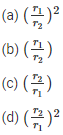Answer: (c) $$\left(\frac{r_{2}}{r_{1}}\right)$$

8. Choose the factor on which the orbital velocity does not depend when the satellite is orbiting close to the earth’s surface
(a) The mass of the earth
(b) The mass of the satellite
(d) The radius of the earth

Answer: (b) The mass of the satellite

9. If a satellite takes time T for revolution, the kinetic energy is proportional to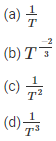Answer: (b) $$T^{\frac{-2}{3}}$$

10. The atmosphere around the earth is held by
(a) Gravity
(b) Winds
(c) Clouds
(d) None of the above

## Kinematics MCQ for NEET

NEET  Physics is the scoring paper in the medical entrance examination. Here, you will discover the NEET Physics MCQ Questions for all Concepts as per the latest syllabus. Practice more on a regular basis with these NEET Physics objective questions on air pollution and improve your subject knowledge & problem-solving skills along with time management. NEET Physics Kinematics Multiple Choice Questions make you feel confident in answering the question in the exam & increases your scores to high.

## MCQ on Kinematics

1. Consider two cars M and N. These cars start from the same point and travel in a straight line such that their positions are xM(t) = at + bt2 and xN(t) = ft – t2. Determine the time at which both the cars are having the same velocity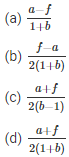Answer: (b) $$\frac{f-a}{2(1+b)}$$

2. Below is the distance-time curve traced by the particle. What is the maximum instantaneous velocity of the particle around the point(a) D
(b) B
(c) A
(d) C

3. Below is the graph of a body whose initial velocity is v0. The body travels through a straight line with acceleration as shown in the graph. Determine the maximum velocity of the body.(a) v0−4at0
(b) v0+4at0
(c) v0+(at0)
(d) $$v_{0}+\frac{9}{2}\left(a t_{0}\right)$$

Answer: (d) $$v_{0}+\frac{9}{2}\left(a t_{0}\right)$$

4. What will be the shape of the x→y graph, if the relation between time and displacement of the moving body is t = 2αx2 where α is constant.
(a) Circle
(b) Straight line
(c) Parabola
(d) Hyperbola

5. Which of the following statement is true if a body moves in a semicircular track whose radius is R
(a) 2R is the displacement of the body
(b) πR is the distance travelled by the body
(c) Both (a) and (b) are correct
(d) None of the above

Answer: (c) Both (a) and (b) are correct

6. What does the area under acceleration-time graph represent for any given time interval
(a) Final velocity
(b) Distance travelled
(c) Change in the velocity in that time interval
(d) Displacement of the particle

Answer: (c) Change in the velocity in that time interval

7. When can we say that the resultant of the two vectors is maximum
(a) When the vectors are acting in the opposite direction
(b) Both the vectors are acting in the same direction
(c) When the vectors are at right angles
(d) When the vectors are parallel to each other

Answer: (b) Both the vectors are acting in the same direction

8. From the below, select the unit vector along $$\hat{i}+\hat{j}$$Answer: (a) $$\frac{\hat{i}+\hat{j}}{\sqrt{2}}$$

9. A particle with radius R is moving in a circular path with constant speed. The time period of the particle is T. Calculate the time for the following after $$t=\frac{T}{6}$$. What is the average velocity of the particleAnswer: (c) $$\frac{6 R}{T}$$

## Physics Thermodynamics MCQ for NEET

NEET  Physics is the scoring paper in the medical entrance examination. Here, you will discover the NEET Physics MCQ Questions for all Concepts as per the latest syllabus. Practice more on a regular basis with these NEET Physics objective questions on air pollution and improve your subject knowledge & problem-solving skills along with time management. NEET Physics Physics Thermodynamics Multiple Choice Questions make you feel confident in answering the question in the exam & increases your scores to high.

## MCQ on Physics Thermodynamics

1. Following is the diagram of the thermodynamic process. Refer the diagram and match the followingP Process – I (a) Adiabatic
Q Process – II (b) Isobaric
R Process – III (c) Isochoric
S Process – IV (d) Isothermal
(a) (P,c), (Q,d), (R,b), (S,a)
(b) (P,c), (Q,d), (R,b), (S,a)
(c) (P,a), (Q,c), (R,d), (S,b)
(d) (P,c), (Q,a), (R,d), (S,b)

Answer: (d) (P,c), (Q,a), (R,d), (S,b)

2. Which of the following process is used to do maximum work done on the ideal gas if the gas is compressed to half of its initial volume?
(a) Isothermal
(b) Isochoric
(c) Isobaric

3. ABCDA is a cyclic process explaining the thermodynamic process. What is the work done by the system in the cycle(a) Zero
(b) $$\frac{\rho_{0} V_{0}}{2}$$
(c) ρ0V0
(d) 2ρ0V0

4. What is the ratio of $$\frac{C_{p}}{C_{v}}$$ for gas if the pressure of the gas is proportional to the cube of its temperature and the process is an adiabatic process.
(a) 2
(b) 3/2
(c) 4/3
(d) 5/3

5. The coefficient performance of a refrigerator is 5. If the temperature inside the freezer is -20℃, calculate the heat rejected to the surrounding
(a) 11℃
(b) 41℃
(c) 21℃
(d) 31℃

6. A thermodynamic system is explained with the help of the graph ABCD. What is the heat rejected by the system during the cycle?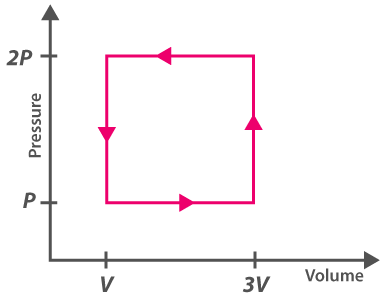(a) pV
(b) 2pV
(c) 4pV
(d) 1/2pV

7. Select the incorrect statement
(a) For the triple point of water, 1°K is equal to 1/273.16.
(b) The first law of thermodynamics is also known as the law of thermal equilibrium
(c) The triple point of water is one of the reference points on the thermodynamic scale of temperature

8. What is a necessary condition for a reaction to be spontaneous at all temperatures?
(a) ΔH < ΔG
(b) ΔG and ΔH should be positive
(c) ΔH = ΔG = 0
(d) ΔG and ΔH should be negative

Answer: (d) ΔG and ΔH should be negative

9. A gas is compressed to half of its initial volume isothermally. The same gas is compressed again until the volume reduces to half through an adiabatic process. Then
(a) Work done during isothermal compression is more
(b) Work done is independent of the processes used for compression
(c) Work done is more during the adiabatic process
(d) Work done is dependent on the atomicity of the gas

10. Select the factor that affects the heat of reaction which is based on Kirchoff’s equation
(a) Molecularity
(b) Temperature
(c) Pressure
(d) Volume

11. For all the reactions what is the nature of the chemical dissociation?
(a) Exothermic
(b) Reversible
(c) Endothermic
(d) Reversible and endothermic

12. Which of the following is the largest unit of energy
(a) Electron volt
(b) Joule
(c) Calorie
(d) Erg

## Atoms and Nuclei MCQs for NEET

NEET  Physics is the scoring paper in the medical entrance examination. Here, you will discover the NEET Physics MCQ Questions for all Concepts as per the latest syllabus. Practice more on a regular basis with these NEET Physics objective questions on air pollution and improve your subject knowledge & problem-solving skills along with time management. NEET Physics Atoms and Nuclei Multiple Choice Questions make you feel confident in answering the question in the exam & increases your scores to high.

## MCQ on Atoms and Nuclei

1. Which of the following should we consider to explain the fine structure of the spectrum of hydrogen?
(a) The presence of neutrons in the nucleus
(b) Orbital Angular Momentum
(c) Infinite size of the nucleus
(d) Spin angular Momentum

2. Which of the following statements is true about Bohr’s atomic model?
(a) The electron can only move in particular orbits only
(b) An electron radiates energy only when it jumps to another orbit
(c) An atom consists of a positively charged nucleus
(d) All of the above

Answer: (d) All of the above

3. If the radius of Bohr’s first orbit is a0, what is the radius of the nth orbit?
(a) na0
(b) a0/n
(c) n2a0
(d) a0/n2

4. Of the various series of the hydrogen spectrum, which of the following lies wholly in the ultraviolet region?
(a) Balmer Series
(b) Paschen Series
(c) Brackett series
(d) Lyman Series

5. In Bohr’s model, if the radius of the first orbit is r0, what is the radius of the third orbit?
(a) 3r0
(b) 9r0
(c) r0/9
(d) r0

6. What is the energy needed to ionize H-atom from its second excited state if the energy of the ground state of H-atom is 13.6 eV?
(a) 3.4 eV
(b) 1.51 eV
(c) 12.1 eV
(d) 13.6 eV

7. Which of the following regions does X-ray lie between?
(a) visible and ultraviolet regions
(c) short radio waves and visible region
(d) gamma rays and ultraviolet region

Answer: (d) gamma rays and ultraviolet region

8. Which of the following is a correct statement?
(a) Equal fractions disintegrate in equal intervals of time.
(b) The rate of disintegration is directly proportional to the number of atoms present in the sample at that instant.
(c) A radioactive sample takes an infinite time to disintegrate completely
(d) All of the above

Answer: (d) All of the above

9. Which of the following is true about gamma rays?
(a) Gamma rays are uncharged high energy rays coming from the outer space
(b) Gamma rays are high energy rays emitted by radioactive elements
(c) Gamma rays are charged particles emitted by radioactive elements
(d) Gamma rays are charged high energy rays coming from the outer space

Answer: (b) Gamma rays are high energy rays emitted by radioactive elements

10. How many numbers of spectral lines are observed when hydrogen atoms are excited from ground state to the state of principal quantum number 4?
(a) 2
(b) 3
(c) 5
(d) 6

## MCQs on Series and Parallel Circuits

NEET  Physics is the scoring paper in the medical entrance examination. Here, you will discover the NEET Physics MCQ Questions for all Concepts as per the latest syllabus. Practice more on a regular basis with these NEET Physics objective questions on air pollution and improve your subject knowledge & problem-solving skills along with time management. NEET Physics Series and Parallel Circuits Multiple Choice Questions make you feel confident in answering the question in the exam & increases your scores to high.

## MCQs on Series and Parallel Circuits

1. A certain circuit is composed of two parallel resistors. The total resistance is 1,403 Ω. One of the resistors is 2 Ω. The other resistor value is
(a) 1,403 Ω
(b) 4.7 kΩ
(c) 2 kΩ
(d) 3,403 Ω

2. A voltage divider consists of ot two 100 kΩ resistors and a 12 V source. What will the voltage be if a load resistor of 1 MΩ is connected to the output?
(a) 0.57 V
(b) 6 V
(c) 12 V
(d) 5.7 V

3. The parallel combination of a 1.5 kΩ resistor and 470 Ω resistor is in series with the parallel combination of five 1 kΩ resistors. The source voltage is 50 V. The percentage of the load current through any single 1 kΩresistor is
(a) 25 %
(b) 20 %
(c) 100 %
(d) 50 %

4. On which of the following voltage range settings will a voltameter present minimum load on a circuit?
(a) 1 V
(b) 50 V
(c) 500 V
(d) 1,000 V

5. A Voltage divider consists of two 68 kΩ resistors and a 24 V source. The unknown output voltage is
(a) 12 V
(b) 24 V
(c) 0 V
(d) 6 V

6. Two 3.3 kΩ resistors are in series combination are in parallel with a 4.7 kΩ resistor. What will be the voltage across the 4.7 kΩ resistors if the voltage across one of the 3.3 kΩ resistors is 12 V
(a) 24 V
(b) 12 V
(c) 0 V
(d) 6 V

7. The 1.2 kΩ resistors are in series and this series combination is in parallel with a 3.3 3.3 kΩ resistor. The total resistance is
(a) 138 Ω
(b) 1,389 Ω
(c) 5,700 Ω
(d) 880 Ω

8. A certain voltage divider consists of three 1 kΩ resistors in series. Which of the following load resistors will have the least effect on the output voltage?
(a) 1 MΩ
(b) 100 kΩ
(c) 1 kΩ
(d) 330 Ω

9. A certain Wheatstone bridge has the following resistor values: R1= 10 kΩ, R2 = 720 Ω and R4 = 2.4 kΩ. The unknown resistance is
(a) 24 Ω
(b) 2.4 Ω
(c) 300 Ω
(d) 3,000 Ω